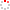#CodeStepByStep## dice_sum

Language/Type: Python random loops
Author: Marty Stepp (on 2019/07/08)

Write a function named `dice_sum` that prompts the user for a desired sum, then repeatedly rolls two six-sided dice until their sum is the desired sum. Here is the expected dialogue with the user:

```Desired dice sum: 9
4 and 3 = 7
3 and 5 = 8
5 and 6 = 11
5 and 6 = 11
1 and 5 = 6
6 and 3 = 9
```

(Because this problem uses random numbers, our test cases check only the general format of your output. You must still examine the output yourself to make sure the answer is correct.)

Type your Python solution code here:

This is a function exercise. Write a Python function as described. Do not write a complete program; just the function(s) above.